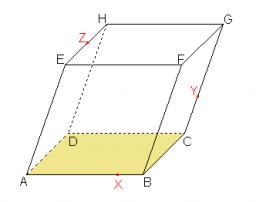# Calculate 4254

The prism's base is a diamond with a side length of 6 cm and a height of 4 cm. The height of the prism is 125% greater than the length of the side of the diamond. Calculate the surface area and volume of the prism.

S =  372 cm2
V =  324 cm3

### Step-by-step explanation:Did you find an error or inaccuracy? Feel free to write us. Thank you!

Tips for related online calculators
Our percentage calculator will help you quickly calculate various typical tasks with percentages.
Do you want to convert length units?
Tip: Our volume units converter will help you convert volume units.

#### Grade of the word problem:

We encourage you to watch this tutorial video on this math problem: